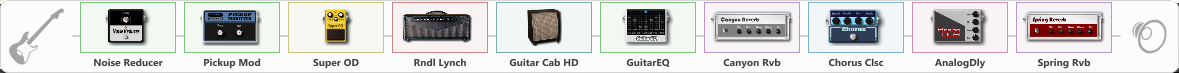# george lynch (dokken) tone

Discussion in 'ToneLib-GFX presets' started by Staspaw15, Jul 24, 2022.

1. george lynch (dokken) tone

Preset name: solo 80's

Effects chain:Effect: "Noise Reducer" (Dynamics / Filter), active - "yes"
{
"Sens" = 85
"Mode" = Soft
}

Effect: "Pickup Mod" (Dynamics / Filter), active - "yes"
{
"Mode" = Single->Humbacker
"Tone" = 50
}

Effect: "Super OD" (Overdrive / Distortion), active - "yes"
{
"Drive" = 43
"Tone" = 82
"Level" = 91
}

Effect: "Rndl Lynch" (Amp simulators), active - "yes"
{
"Gain" = 69
"Bass" = 65
"Middle" = 27
"Treble" = 39
"Presence" = 68
"Master" = 83
"Level (dB)" = 0
}

Effect: "Guitar Cab HD" (Cabinets), active - "yes"
{
"Model" = Soldano Slant (4x12")
"Mic Model" = Dynamic MD421
"Mic Position" = Center
"Mic Distance" = Near
"Low Cut (Hz)" = 80
"Hi Cut (kHz)" = 20.0
"Mix" = 100
"Level (dB)" = 3
}

Effect: "GuitarEQ" (Dynamics / Filter), active - "yes"
{
"160 Hz" = 1
"400 Hz" = -1
"800 Hz" = 0
"1.6 kHz" = 0
"3.2 kHz" = 0
"6.4 kHz" = 2
"12 kHz" = -3
"Level (dB)" = 0
}

Effect: "Canyon Rvb" (Reverberation), active - "yes"
{
"Time" = 9.3
"PreDelay" = 71
"LoDamp" = 0
"HiDamp" = 82
"Mix" = 65
}

Effect: "Chorus Clsc" (Modulation / Sfx), active - "yes"
{
"Speed" = 5.4
"Depth" = 45
"Center" = 4.7
"Mode" = Mono
}

Effect: "AnalogDly" (Delay), active - "yes"
{
"Time" = 380
"Feedback" = 34
"Tone" = 41
"Mix" = 65
}

Effect: "Spring Rvb" (Reverberation), active - "yes"
{
"Time" = 7.0
"PreDelay" = 44
"LoDamp" = 65
"HiDamp" = 62
"Mix" = 59
}

Note: You will need to download and install the ToneLib-GFX software to use the preset.

#### Attached Files:

• ###### george_lynch_dokken_tone.tlgfx
File size:
924 bytes
Views:
1,682
Onur likes this.